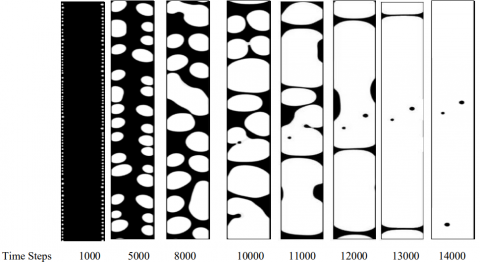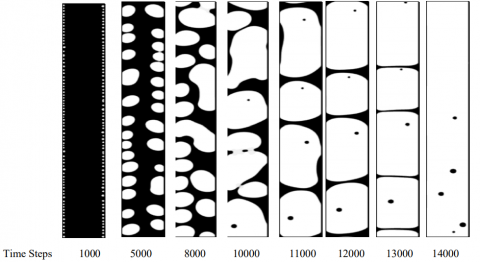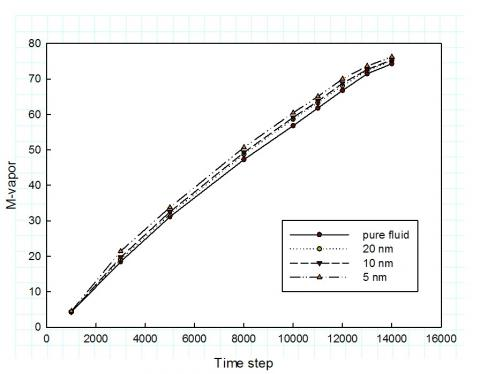# Analysis of Nanofluids Phase Transition in Pipe Using the Lattice Boltzmann Method

Analysis of Nanofluids Phase Transition in Pipe Using the Lattice Boltzmann Method

ShouGuang Yao* XinWang Jia AnJie Hu RongJuan Li

School of Energy and Power Engineering, JiangSu University of Science and Technology, Zhenjiang, China

key Laboratory of Low-grade Energy Utilization Technologies & Systems, Ministry of Education, Chong Qing University, ChongQing, China;

School of Energy and Civil Engineering, Harbin University of Commerce, Harbin, China.

Corresponding Author Email:
zjyaosg@126.com
Page:
103-108
|
DOI:
https://doi.org/10.18280/ijht.330217
N/A
|
Accepted:
N/A
|
Published:
30 June 2015
| Citation

OPEN ACCESS

Abstract:

In this paper, a lattice Boltzmann model is applied to simulate the phase transition of nanofluids. The phase transition in this model is achieved by an interparticle pseudo-potential, which is based on the brown motion of nanoparticles in the base fluid. Using this model, the vapor-liquid flow pattern maps are obtained, and the mass change of vapor and liquid phases is also analyzed. The simulation results show that heat transfer ability of nanofluids is enhanced with particles. However, with the nanoparticle size increasing, the heat transfer ability of nanofluids becomes weak. These results agree well with experimental results.

Keywords:

Nanofluids, Lattice Boltzmann method, Phase transition, Flow pattern maps.

1. Introduction

Due to their high thermal conductivity, the nanofluids have extensive application potential in terms of strengthening heat transfer . To study the heat transfer of nanofluids, lots of experiment research have been done in recent years: Ahmad et al  have shown that nanoparticles can strengthen the convective heat transfer in laminar flow under a constant heat flux; Sarit and Kedzierski et al. [3, 4] got the similar results that the nanoparticles can greatly improve the heat transfer ability; Zhang et al.  farther investigated the influence of particle size, and discovered that the smaller the particle size, the higher heat transfer coefficient. The influence of nanoparticles on the nucleate boiling was also studied by researchers. However, Bang et al.  found that, instead of improving the heat transfer, the nanoparticles deteriorated the heat transfer in nucleate boiling.

Besides of experiment research, numerical simulation has been applied to study the detail of the properties of nanofluids in these years: Xiao et al.  found that the heat transfer capability of nanofluids is much stronger compared with the pure fluid, they also pointed out that the smaller the diameter of nanoparticles, the more effective the heat transfer; Xuan’s considered the influence of the interaction potential between particles and brown force, and also found that the Brownian motion of nanofluids can enhance the energy transfer.

In these simulation, since the base fluid is single phase, nanofluids are generally considered as homogeneous fluids in

the analysis of heat transfer enhancement, namely the parameters of nanofluids are obtained from the parameters of nanoparticles and base liquid. However, for a two-phase medium, there are interphase drag force, brown force, force caused by potential energy, gravity and other complex forces between the nanoparticles and base fluids, which makes the fluid system much complex. In this case, the nanofluids cannot be considered as homogeneous fluid, and homogeneous model is no longer suitable for the nanofluids with two-phase medium .

To numerically investigate the influence of nanoparticles on the two-phase fluid, we can add the nanoparticles’ influence in a multiphase numerical model. The method to simulate two-phase fluid we applied here is lattice Boltzmann method. Compare with traditional numerical method, it has many advantages in multiphase simulation such as simple implementation, good concurrency, and simple boundary treatment, etc.

In this paper, a nanofluids phase change model is established based on the lattice Boltzmann method, and phase change process of nanofluid in a vertical tube is simulated. In this simulation, the multi-component LBM model  and the S-C model  are applied. Then simulation results are compared with available results in references . The mechanism of nanofluid heat transfer enhancement is also explained based on the results.

2. Numerical Simulations

2.1 Lattice Boltzmann model for nanofluids

The evolution equations for nanofluids :

$f_{i}^{\sigma}\left(\mathbf{x}+\mathbf{e}_{i} \Delta t, t+\Delta t\right)-f_{i}^{\sigma}(\mathbf{x}, t)=\left(f_{i}^{\sigma e q}(\mathbf{x}, t)-f_{i}^{\sigma}(\mathbf{x}, t)\right) / \tau^{\sigma}$    (1)

where $\tau^{\sigma}$ is the dimensionless collision-relaxation time constant of the$\sigma$ th component of the fluid,$\mathbf{e}_{\mathbf{i}}$ is the lattice velocity vector, the subscript i represents the lattice velocity direction, and $f_{i}^{\sigma}(\mathbf{x}, t)$ is the population of the particles of $\sigma$ th component with velocity $\mathbf{e}_{\mathbf{i}}$ at lattice $x$ and time t. The nanofluid is a suspension consisting of nanoparticles and a base liquid, $\sigma=1,2$ ,$f_{i}^{\sigma e q}(\mathbf{x}, t)$ is the equilibrium distribution function which should be carefully selected. Corresponding to the D2Q9 model, the single-particle distribution function in the equilibrium state is given as:

$f_{i}^{\sigma e q}=\omega_{i} f^{\sigma}\left[1+3 \frac{\mathbf{e}_{i} \cdot \mathbf{u}_{i}^{\sigma e q}}{c^{2}}+4.5 \frac{\left(\mathbf{e}_{i} \cdot \mathbf{u}_{i}^{\sigma e q}\right)^{2}}{c^{4}}-1.5 \frac{\mathbf{u}_{i}^{\sigma e q} \cdot \mathbf{u}_{i}^{\sigma e q}}{c^{2}}\right]$     (2)

where $\mathcal{W}_{i}$ is the weight coefficient. $f^{\sigma}=\sum_{i} f_{i}^{\sigma}$ is the local number density of the $\sigma$ th component, the mass density and velocity of the $\sigma$ th component can be expressed as:

$\rho_{\sigma}=m_{\sigma} f^{\sigma}, \rho_{\sigma} \mathbf{u}_{\sigma}=m_{\sigma} \sum_{i} f_{i}^{\sigma} \mathbf{e}_{i}$   (3)

where $m_{\sigma}$ is the molecular mass of the $\sigma$ th component.

As previously mentioned, the nanofluid is a colloidal suspension consist of nanoparticles and the base fluid. Since the diameter of nanoparticles are much smaller than the lattice length, the shape of the nanoparticles is neglected, only their force influence is considered. We assumed $F_{\sigma}$ is the vector sum of all forces acting on the $\sigma$ th component in per unit lattice volume. For nanoparticles, $F_{\sigma}$ includes buoyancy, gravitational force, Brownian force, drag force and dispersion force resulting from the repulsive potential. For the base fluid, $F_{\sigma}$ is mainly composed of the reaction of drag force and Brownian force. These forces are given as follows:

(1) Drag force :

$F_{D}=-6 \pi \mu r_{p} \Delta u$    (4)

where $\mu$ is the viscosity of the fluid, and $\Delta u$ is the velocity difference between the particle and the fluid, $r_{p}$ is the radius of the suspended nanoparticle.

(2) Brownian force :

$F_{B}=\zeta \sqrt{\frac{1}{D} \frac{2 k_{B}^{2} T^{2}}{\Delta t}}$     (5)

where $k_{B}$ is the Boltzmann constant, and T is the absolute temperature of the fluid, D is the diffusion coefficient of nanoparticles, $\Delta t$ is the time step of Gaussian movement.

The forces acting on the fluid molecules in a given lattice can be expressed as the reaction of the drag force and Brownian force:

$F=-\left(F_{D}+F_{B}\right)$     (6)

2.2 Potential force term for nanofluid

In order to simulate phase change, the potential force must be considered. For the pseudopotential model, the pseudopotential function is given as follows :

$\mathbf{V}_{\sigma \bar{\sigma}}\left(\mathbf{x}, \mathbf{x}^{\prime}\right)=G_{\sigma \bar{\sigma}}\left(\mathbf{x}, \mathbf{x}^{\prime}\right) \phi_{\sigma}\left(f^{\sigma}(\mathbf{x})\right) \phi_{\sigma}\left(f^{\bar{\sigma}}\left(\mathbf{x}^{\prime}\right)\right)$   (7)

where $\phi_{\sigma}\left(f^{\sigma}(\mathbf{x})\right)$ and $\phi_{\sigma}\left(f^{\bar{\sigma}}\left(\mathbf{x}^{\prime}\right)\right)$ are the effective densities at position x for $\sigma$ th component, and at $\mathbf{x}^{\prime}=\mathbf{x}+\mathbf{e}_{i}$ for$\bar{\sigma}$th component respectively.

If only considering the isotropic interaction between neighboring nodes, then Green function can be expressed as:

$\left\{\begin{array}{l}G_{\sigma \bar{\sigma}}\left(\mathbf{x}, \mathbf{x}^{\prime}\right)=G_{\sigma \bar{\sigma}}\left|\mathbf{x}-\mathbf{x}^{\prime}\right|=1 \\ G_{\sigma \bar{\sigma}}\left(\mathbf{x}, \mathbf{x}^{\prime}\right)=0 \quad\left|\mathbf{x}-\mathbf{x}^{\prime}\right|=\sqrt{2} \\ G_{\sigma \bar{\sigma}}\left(\mathbf{x}, \mathbf{x}^{\prime}\right)=G_{\sigma \bar{\sigma}} / 4 \text { Others }\end{array}\right.$    (8)

where $G_{\sigma \bar{\sigma}}\left(\mathbf{x}, \mathbf{x}^{\prime}\right)=G_{\overline{\sigma \sigma}}\left(\mathbf{x}^{\prime}, \mathbf{x}\right)$ is the interaction strength between $\sigma$ th and $\bar{\sigma}$ th component. Then the particle interactions of $\sigma$ th component can be chosen as:

$F_{\sigma}(\mathbf{x})=-\phi\left(f^{\sigma}(\mathbf{x})\right) \sum_{x} \sum_{\sigma} G_{\sigma \bar{\sigma}}\left(\mathbf{x}, \mathbf{x}^{\prime}\right) \phi_{\sigma}\left(f^{\bar{\sigma}}\left(\mathbf{x}^{\prime}\right)\right)\left(\mathbf{x}-\mathbf{x}^{\prime}\right)$    (9)

In a false potential model, the influence of the interaction force between particles is reflected by changing the equilibrium velocity in the equilibrium distribution function.   The new equilibrium velocity is given by:

$\rho_{\sigma} \mathbf{u}_{\sigma}^{\mathrm{eq}}=\rho_{\sigma} \mathbf{u}^{\prime}+0.5 \tau_{\sigma} \mathbf{F}_{\sigma}$  (10)

To ensure the collision momentum conservation, the following relation must be satisfied:

$\mathbf{u}^{\prime}=\sum_{\sigma}\left(\rho_{\sigma} \mathbf{u}_{\sigma} / \tau_{\sigma}\right) / \sum_{\sigma}\left(\rho_{\sigma} / \tau_{\sigma}\right)$     (11)

The real speed can is given by:

$\rho \mathbf{U}=\sum_{\sigma} \rho_{\sigma} \mathbf{u}^{\prime}+0.5 \sum_{\sigma} \tau_{\sigma} \mathbf{F}_{\sigma}$    (12)

In case of nanofluid, the forces acting on the base fluid include: resistance, brown force and repulsive force between the molecules. So the Eq. (10) can be written as :

$\rho_{\sigma} \mathbf{u}_{\sigma}^{\mathrm{eq}}=\rho_{\sigma} \mathbf{u}^{\prime}+0.5 \tau_{\sigma} \mathbf{F}^{\sigma}$    (13)

where $F^{\sigma}$ is the sum of the force vectors in the unit volume grid.

$\sigma=1$ ,$F^{\sigma}$ represents the force acting on base fluid, including: resistance, brown force, repulsive force between the molecules and gravity.

$\sigma=2$ ,$F^{\sigma}$ represents the force acting on vapor, including: force between the molecules and gravity.

3. Results and Discussion

To investigate the phase transition in the vertical tube under the constant heat flux, the modified lattice Boltzmann model is applied to simulate the process of phase change in isotheral condition. The simulation system is in a range of 100×800 lattice sites.

In the simulation, the density given by $\rho_{0}=8$ , the relaxation time is chosen as $\tau=1$, and the gravitational acceleration is given by $g=0.00003$ . All physical quantities is dimensionless. Periodic boundary scheme is applied on upper and lower boundaries, while the left and right boundaries are the bounce-back boundary scheme. Initially, the area was filled with liquid. In order to enable the equation to be evolving, the heat was given a disturbance of 1%. Figs.1, 2, 3, 4 are the vapor- liquid two phase diagrams of pure liquid and nanofluid with different particle diameter, respectively. In these figures, the white part is the vapor, meaning the formation of bubbles; and black part is the liquid. As we can see in these figures, the process of the bubble growth for all these conditions are similar: small bubbles begin to form on the walls firstly, and then the small bubbles continuous integrate into large bubbles. The hollow bubble rate in the whole area is increasing.

## 1.pngFigure 1. Pure liquid

## 2.pngFigure 2. Nanofluid with particle size of 5 nm

## 3.pngFigure 3. Nanofluid with particle size of 10 nm

## 4.pngFigure 4. Nanofluid with particle size of 20 nm

In the case of pure fluid (fig. 1), the entire changing processes of flow patterns are similar with flow patterns in the heating vertical pipe, since it also experienced the bubble flow (1000-10000), the slug flow (11000), the mass flow (12000-13000) and mist flow (14000). Bubble flow : there is a continuous liquid phase, and the gas phase is dispersed as bubble within the liquid continuum. The bubble travel with a complex motion within the flow may be coalescing and are generally of non-uniform size; Slug flow: This flow regime occurs when the bubble size tends toward that of the channel diameter and characteristic bullet-shaped bubbles are formed; Mass flow: interrupted liquid phase with chaotic gas distribution; Mist flow: in which the velocity of the continuous gas phase is so high that it reaches as far as the tube wall and entrains the liquid in the form of droplets.

Vapor-liquid flow patterns of nanofluid mainly are bubble flow and the massive flow, which is different from that of pure liquid from fig.2, 3, 4. This phenomenon is the same as the experimental results of Ref.. The reason is that the motion state and bubble layer structure of thermal boundary layer near heating surface have been changed, because of the addition of nanoparticles.

## 5.jpgFigure 5. The effect of particle diameters on liquid mass

## 6.jpgFigure 6. The effect of particle diameters on vapor mass

To further investigate the influence of particle size on the boiling process, we also compared the liquid mass and the vapor mass evolution based on the simulation results. The effect of particle diameters on the liquid mass and vapor mass are shown in Fig. 5 and Fig. 6, respectively. By comparing Fig.5 with Fig.6: we can find that for the same time step, the quality of vapor in the nanofluid is higher than that of pure liquid. Besides under the same condition, the smaller the diameter of nanoparticles, the higher the vapor mass produced. These results show that the heat capacity of nanofluid is better than that of pure fluid, and with the increase of nanoparticle diameter, heat effect will be gradually weaker. The reason is that the physical properties of working mediums are changed by the addition of the nanoparticles, result in the ability of thermal conductivity being strengthened, and the formation and growth of bubbles on the heating surface are also improved. So boiling heat transfer is enhanced . At the same time, the disturbance in the thermal boundary layer near the heating surface is enhanced by the Brown motion. Hence, as the particle diameter decreases, Brown motion tends to be fierce, resulting in heat transfer increasing.

4. Conclusions

This paper established a lattice Boltzmann model which is suitable for simulation of phase change process of the nanofluid, and the reaction of nanoparticles on liquid phase was numerically investigated. Some conclusions can be obtained based on the simulation results:

(1) As a new working medium, the heat transfer ability of nanofluid is stronger than that of pure liquids;

(2) With the increase of the nanoparticle size, the heat transfer ability of nanofluids become weak.

This article explains the heat transfer mechanism of the experiments in Ref. [12,17], and the proposed model is verified to be able to simulate heat transfer with phase change of nanofluids. However, quantitative comparison and analysis with experiments are still need to be further studied, and the particles are treated as points with the shape ignored in this article, so there is a certain deviation with actual situation.

Acknowledgement

This work was supported by the National Natural Science Foundation of China (Grant No. 51176069).

References

1. Wang X Q and Mujumdar A S, Heat transfer characteristics of nanofluids: a review, Int. J. Therm. Sci, vol.46, pp. 1-19, 2007.

2. Ahmad A, Mansour K, Masoud D and Masoud R, An Experimental Study on Nanofluids Convective Heat Transfer Through a Straight Tube under Constant Heat Flux, Chinese J Chem Eng, vol.10, pp.1082-1088, 2013.

3. Sarit K D and Nandy P and Wilfried R, Pool boiling characteristics of nano-fluids, Int. J. Heat mass Transf, vol.46, pp. 851-862, 2003.

4. Kedzierski M A and Gong M, Effect of CuO nanolubricant on R134a pool boiling heat transfer, Int. J. Refri, vol.5, pp. 791-799, 2009.

5. Zhang Shaobo, Luo Zhongyang, Shou Chunhui, Ni Mingjiang and Cen Kefa, Heat Transfer Properties of CuO-water Nanofluids in Laminar Flow. Proceedings of the CSEE, vol.29, pp. 58-65, 2009.

6. Bang I C and Chang S H, Boiling heat transfer performance and phenomena of Al2O3-water nanofluids from a plain surface in a pool. Int. J. Heat mass Transf, vol.48, pp. 2407-2419, 2005.

7. Xiao Baolan, Yu Xiaoli, Zhong Xun, Experimental and numerical study of nanofluid flow And heat transfer performance. Journal of Zhejiang University, vol.44, pp. 1149-1154, 2010.

8. Xuan Y and Li Q, Heat transfer enhancement of nanofluids, Int.J. Heat Fluid Flow, vol.21, pp.58, 2000.

9. Qi Cong,Yan Shengnan and Tian Yurong, Rectangular cavity nano fluid two-phase lattice Boltzmann simulation of natural convection, Chinese Society of Engineering Thermophysics, vol.35, pp. 282-286, 2014.

10. Mohammad Abu Taher, Yeon Won Lee and Heuy Dong Kim, Heat Transfer Enhancement of Cu-H2O Nanofluid with Internal Heat Generation Using LBM, Open Journal of Fluid Dynamics, vol.3, pp. 92-99, 2013.

11. X. Shan and H. Chen, Lattice Boltzmann model for simulating flows with multiple phases and components, Phys. Rev. E, vol.47, pp.1815, 1993.

12. Xu Li, Li Yuxiu, Xu Jinliang and Liu Guohua, Flow boling heat transfer performance of nanofluids in a micro-channel, Journal of Chemical Engineering of Chinese Universities, vol.4, pp. 559 -564, 2011.

13. Zhao Kai, Li Qiang and Xuan Yimin, Simulation of phase transition with lattice Boltzmann method, Chinese Journal of Computational Physics, vol.25, pp. 151-155, 2008.

14. Zhang Kai, Yu Mingzhou and Lin Jianzhong, Research on the deposition efficiency of nanoparticles in human airways, Journal of China University of Metrology, vol.21, pp. 87-91, 2010.

15. Cui Tengfei, Xuan Yimin and Li Qiang, Simulation of enhancement of mass transfer in nano-fluids by lattice Boltzmann method, CIESC Journal, vol.63, pp.41-46, 2012.

16. Guo Liejin, Two phase and multiphase flow mechanics, Xi’An Jiao Tong University Press, Xi’An2003.

17. Zhao Yanbin and Shi Mingheng, Experimental research on boiling heat transfer of nm sized solid suspension

particles from strengthening pool, Energy Research and Utilization, vol.3, pp. 18-20, 2001.